# AP Chemistry Review Big Idea 1 Big Idea

• Slides: 57AP Chemistry Review Big Idea 1Big Idea #1 “ Atoms, Elements and the Building Blocks of Matter” Basic concepts covered: • Chemical elements are fundamental building blocks of matter. • Matter can be understood in terms of arrangement of atoms. • Atoms retain their identity in chemical reactions.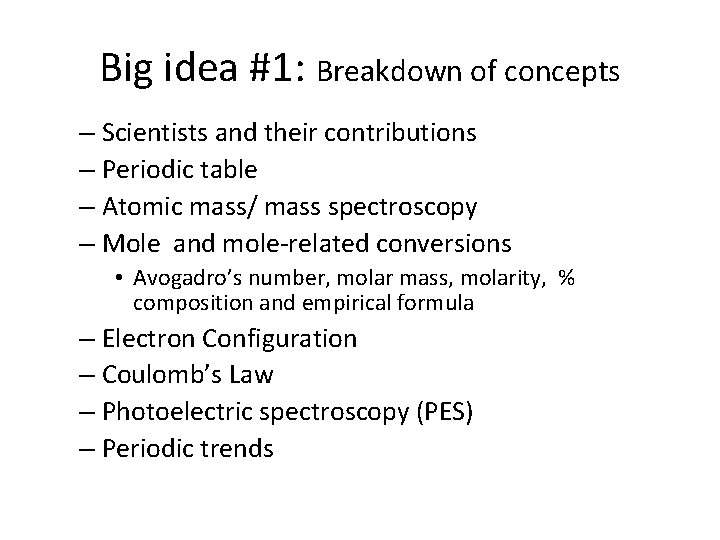Big idea #1: Breakdown of concepts – Scientists and their contributions – Periodic table – Atomic mass/ mass spectroscopy – Mole and mole-related conversions • Avogadro’s number, molar mass, molarity, % composition and empirical formula – Electron Configuration – Coulomb’s Law – Photoelectric spectroscopy (PES) – Periodic trendsThe Electron • Streams of negatively charged particles were found to emanate from cathode tubes. • J. J. Thompson is credited with their discovery (1897). • Thompson measured the charge/mass ratio of the electron to be 1. 76 108 coulombs/g. © 2009, Prentice-Hall,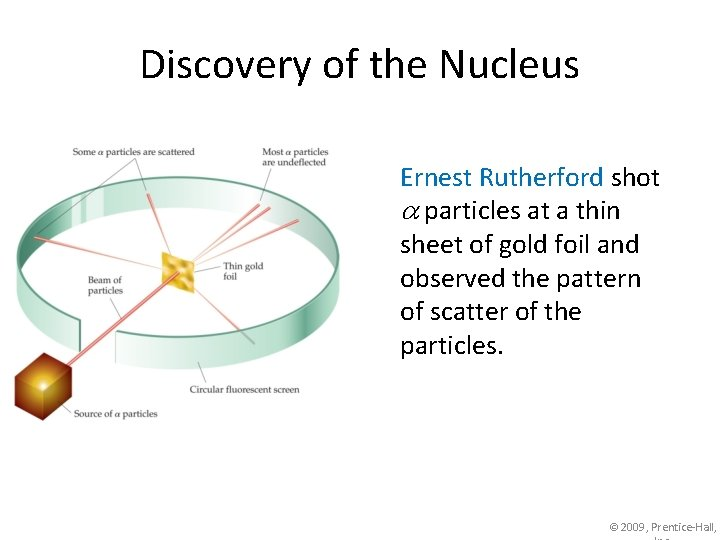Discovery of the Nucleus Ernest Rutherford shot particles at a thin sheet of gold foil and observed the pattern of scatter of the particles. © 2009, Prentice-Hall,The Nuclear Atom • Rutherford postulated a very small, dense nucleus with the electrons around the outside of the atom. • Most of the volume of the atom is empty space. © 2009, Prentice-Hall,The Quantum Mechanical Model • According to quantum theory, it’s impossible to know the exact position and momentum of an electron at the same time. This is known as the Uncertainty Principle.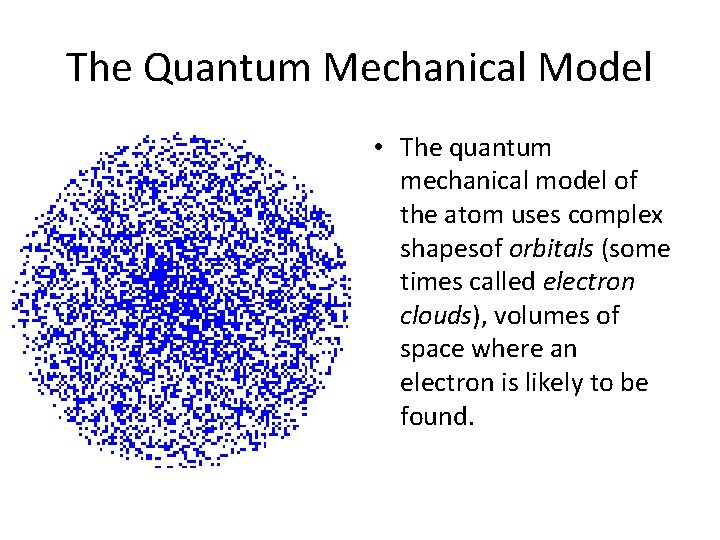The Quantum Mechanical Model • The quantum mechanical model of the atom uses complex shapesof orbitals (some times called electron clouds), volumes of space where an electron is likely to be found.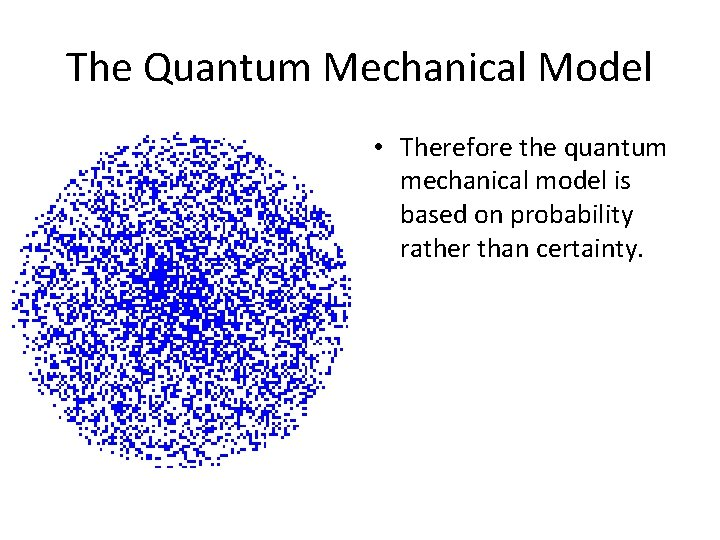The Quantum Mechanical Model • Therefore the quantum mechanical model is based on probability rather than certainty.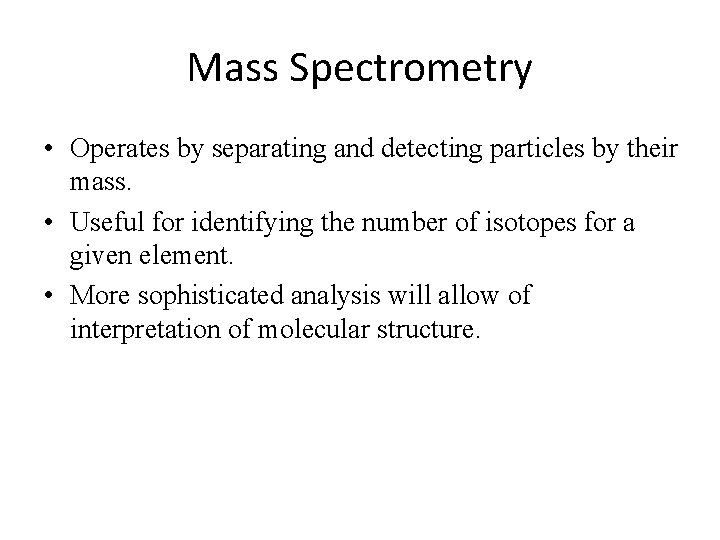Mass Spectrometry • Operates by separating and detecting particles by their mass. • Useful for identifying the number of isotopes for a given element. • More sophisticated analysis will allow of interpretation of molecular structure.Masses of Isotopes determined with a mass spectrometer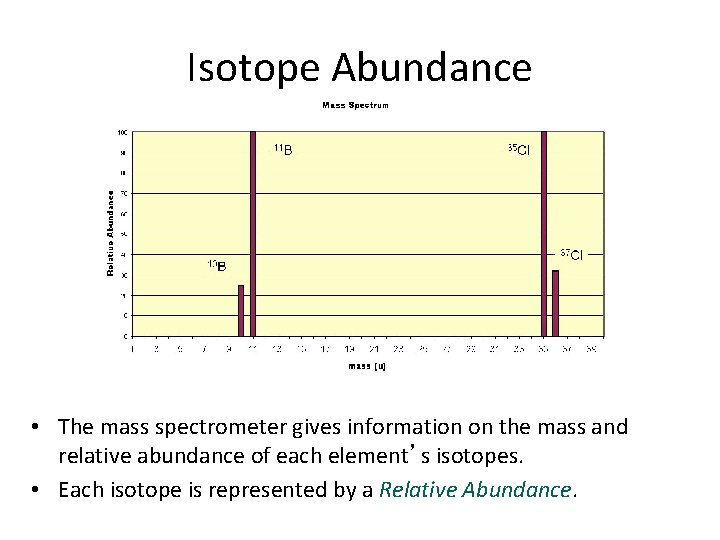Isotope Abundance • The mass spectrometer gives information on the mass and relative abundance of each element’s isotopes. • Each isotope is represented by a Relative Abundance.Interpreting Mass Spectra Calculate the Average atomic mass for Si:Interpreting Mass Spectra = 25. 80 = 1. 39 = 0. 93 Calculate the Average atomic mass for Si: 28. 12 amu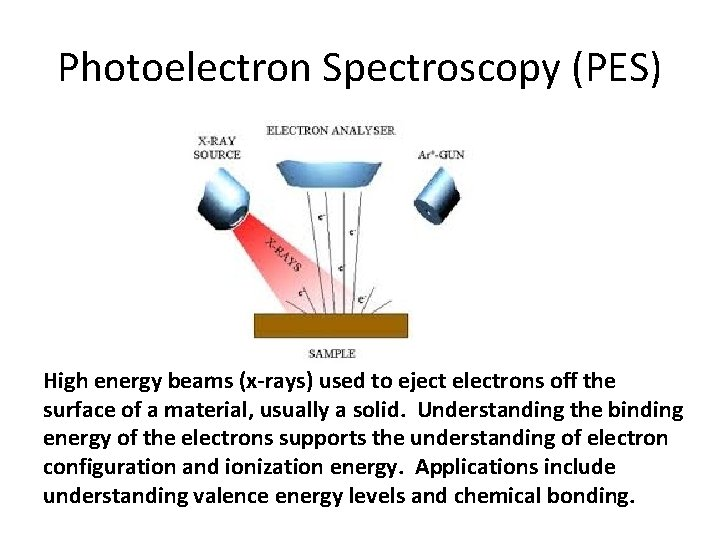Photoelectron Spectroscopy (PES) High energy beams (x-rays) used to eject electrons off the surface of a material, usually a solid. Understanding the binding energy of the electrons supports the understanding of electron configuration and ionization energy. Applications include understanding valence energy levels and chemical bonding.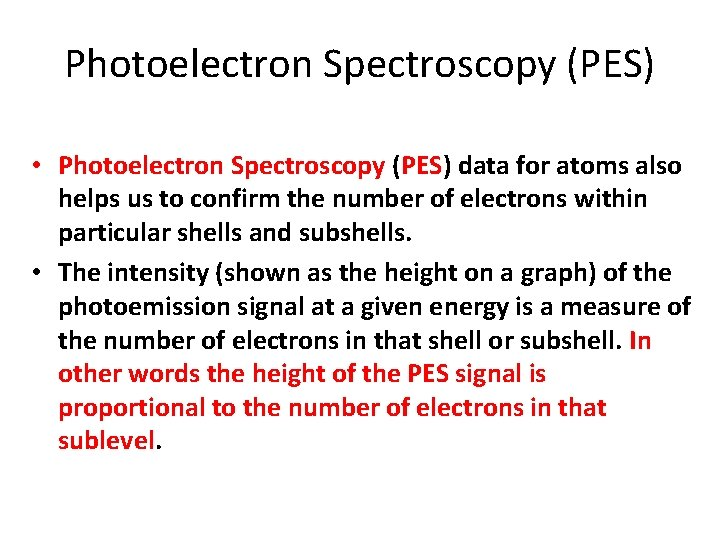Photoelectron Spectroscopy (PES) • Photoelectron Spectroscopy (PES) data for atoms also helps us to confirm the number of electrons within particular shells and subshells. • The intensity (shown as the height on a graph) of the photoemission signal at a given energy is a measure of the number of electrons in that shell or subshell. In other words the height of the PES signal is proportional to the number of electrons in that sublevel.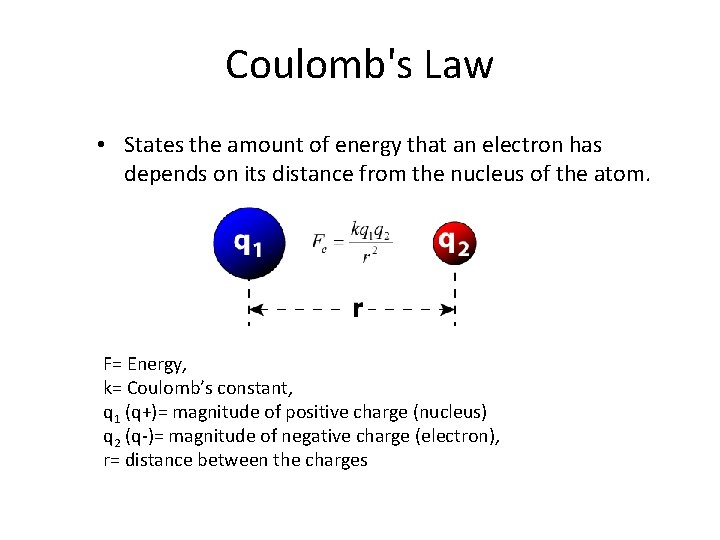Coulomb's Law • States the amount of energy that an electron has depends on its distance from the nucleus of the atom. F= Energy, k= Coulomb’s constant, q 1 (q+)= magnitude of positive charge (nucleus) q 2 (q-)= magnitude of negative charge (electron), r= distance between the charges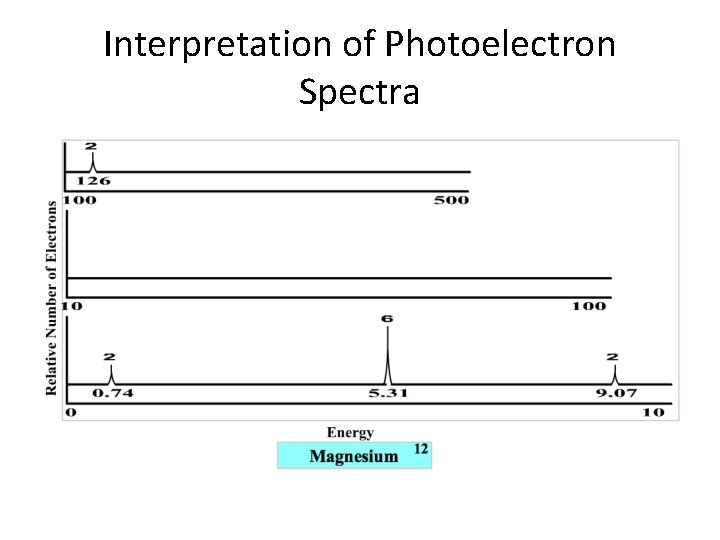Interpretation of Photoelectron Spectra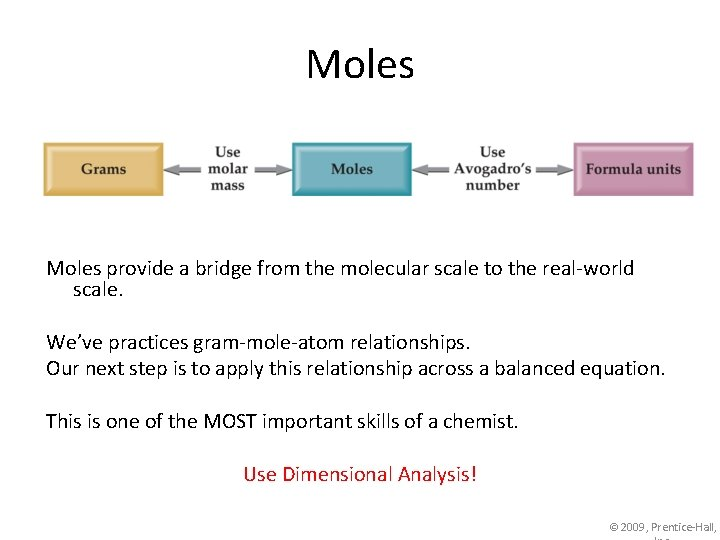Moles provide a bridge from the molecular scale to the real-world scale. We’ve practices gram-mole-atom relationships. Our next step is to apply this relationship across a balanced equation. This is one of the MOST important skills of a chemist. Use Dimensional Analysis! © 2009, Prentice-Hall,Determining the Formula of a Hydrocarbon by Combustion • Combustion involves the addition oxygen to another element. • When hydrocarbon molecules burn completely, the products are always carbon dioxide gas and water.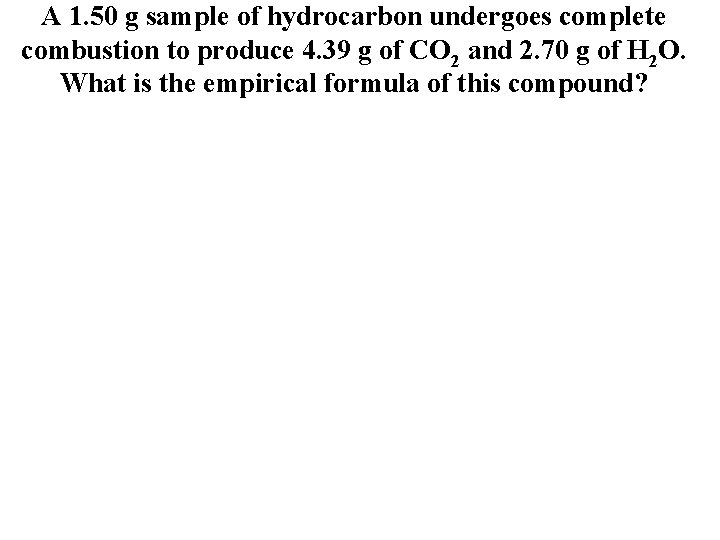A 1. 50 g sample of hydrocarbon undergoes complete combustion to produce 4. 39 g of CO 2 and 2. 70 g of H 2 O. What is the empirical formula of this compound?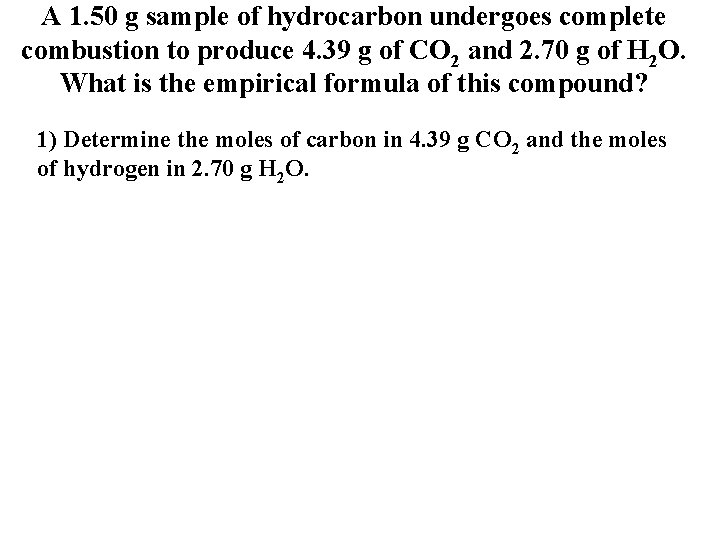A 1. 50 g sample of hydrocarbon undergoes complete combustion to produce 4. 39 g of CO 2 and 2. 70 g of H 2 O. What is the empirical formula of this compound? 1) Determine the moles of carbon in 4. 39 g CO 2 and the moles of hydrogen in 2. 70 g H 2 O.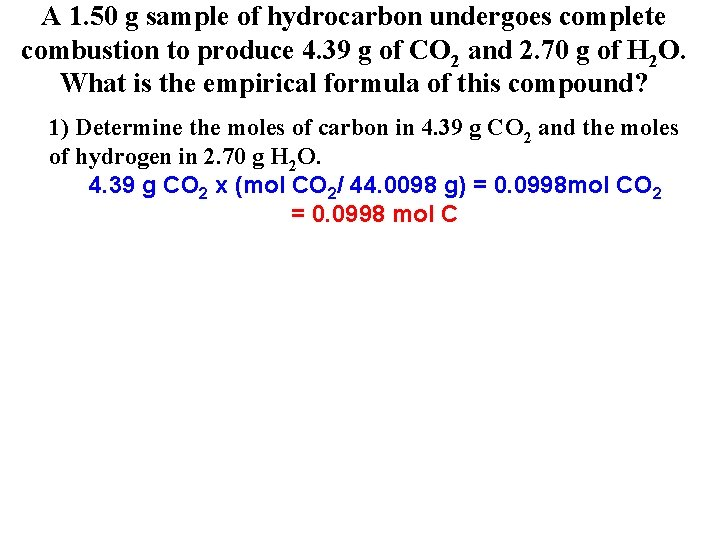A 1. 50 g sample of hydrocarbon undergoes complete combustion to produce 4. 39 g of CO 2 and 2. 70 g of H 2 O. What is the empirical formula of this compound? 1) Determine the moles of carbon in 4. 39 g CO 2 and the moles of hydrogen in 2. 70 g H 2 O. 4. 39 g CO 2 x (mol CO 2/ 44. 0098 g) = 0. 0998 mol CO 2 = 0. 0998 mol C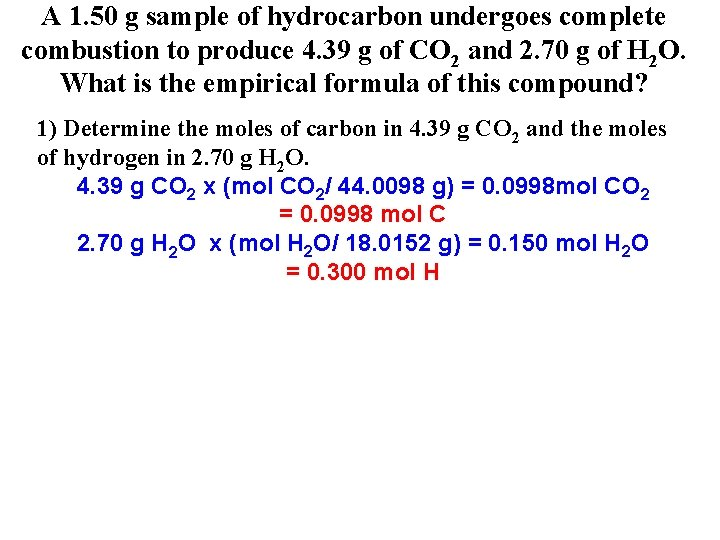A 1. 50 g sample of hydrocarbon undergoes complete combustion to produce 4. 39 g of CO 2 and 2. 70 g of H 2 O. What is the empirical formula of this compound? 1) Determine the moles of carbon in 4. 39 g CO 2 and the moles of hydrogen in 2. 70 g H 2 O. 4. 39 g CO 2 x (mol CO 2/ 44. 0098 g) = 0. 0998 mol CO 2 = 0. 0998 mol C 2. 70 g H 2 O x (mol H 2 O/ 18. 0152 g) = 0. 150 mol H 2 O = 0. 300 mol H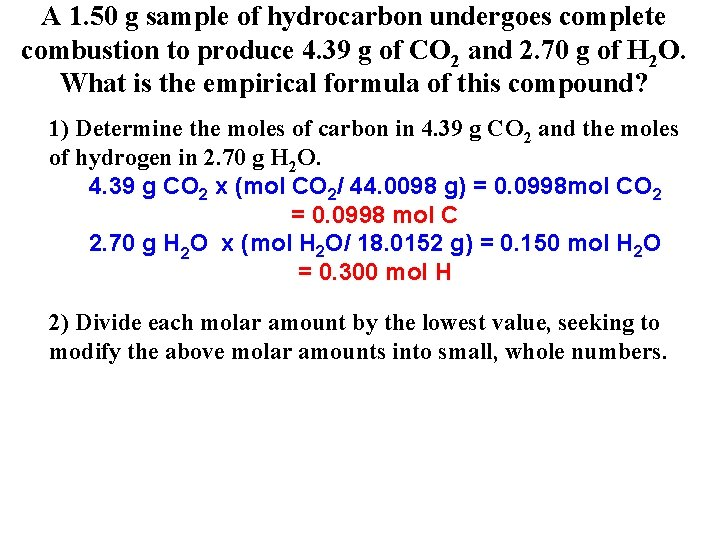A 1. 50 g sample of hydrocarbon undergoes complete combustion to produce 4. 39 g of CO 2 and 2. 70 g of H 2 O. What is the empirical formula of this compound? 1) Determine the moles of carbon in 4. 39 g CO 2 and the moles of hydrogen in 2. 70 g H 2 O. 4. 39 g CO 2 x (mol CO 2/ 44. 0098 g) = 0. 0998 mol CO 2 = 0. 0998 mol C 2. 70 g H 2 O x (mol H 2 O/ 18. 0152 g) = 0. 150 mol H 2 O = 0. 300 mol H 2) Divide each molar amount by the lowest value, seeking to modify the above molar amounts into small, whole numbers.A 1. 50 g sample of hydrocarbon undergoes complete combustion to produce 4. 39 g of CO 2 and 2. 70 g of H 2 O. What is the empirical formula of this compound? 1) Determine the moles of carbon in 4. 39 g CO 2 and the moles of hydrogen in 2. 70 g H 2 O. 4. 39 g CO 2 x (mol CO 2/ 44. 0098 g) = 0. 0998 mol CO 2 = 0. 0998 mol C 2. 70 g H 2 O x (mol H 2 O/ 18. 0152 g) = 0. 150 mol H 2 O = 0. 300 mol H 2) Divide each molar amount by the lowest value, seeking to modify the above molar amounts into small, whole numbers. carbon: 0. 0998 mol C/ 0. 0998 mol = 1 C hydrogen: 0. 300 mol H/ 0. 0998 mol = 2. 9986 = 3 H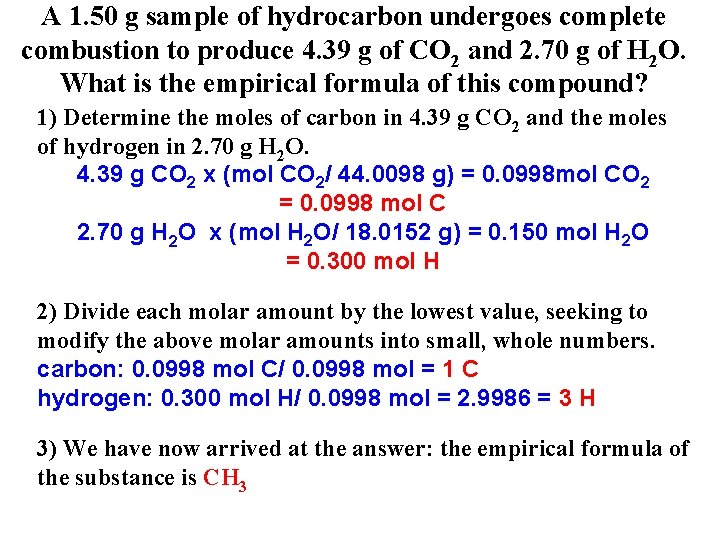A 1. 50 g sample of hydrocarbon undergoes complete combustion to produce 4. 39 g of CO 2 and 2. 70 g of H 2 O. What is the empirical formula of this compound? 1) Determine the moles of carbon in 4. 39 g CO 2 and the moles of hydrogen in 2. 70 g H 2 O. 4. 39 g CO 2 x (mol CO 2/ 44. 0098 g) = 0. 0998 mol CO 2 = 0. 0998 mol C 2. 70 g H 2 O x (mol H 2 O/ 18. 0152 g) = 0. 150 mol H 2 O = 0. 300 mol H 2) Divide each molar amount by the lowest value, seeking to modify the above molar amounts into small, whole numbers. carbon: 0. 0998 mol C/ 0. 0998 mol = 1 C hydrogen: 0. 300 mol H/ 0. 0998 mol = 2. 9986 = 3 H 3) We have now arrived at the answer: the empirical formula of the substance is CH 3A 1. 50 g sample of hydrocarbon undergoes complete combustion to produce 4. 39 g of CO 2 and 2. 70 g of H 2 O. What is the empirical formula of this compound? 1) Determine the moles of carbon in 4. 39 g CO 2 and the moles of hydrogen in 2. 70 g H 2 O. 4. 39 g CO 2 x (mol CO 2/ 44. 0098 g) = 0. 0998 mol CO 2 = 0. 0998 mol C 2. 70 g H 2 O x (mol H 2 O/ 18. 0152 g) = 0. 150 mol H 2 O = 0. 300 mol H 2) Divide each molar amount by the lowest value, seeking to modify the above molar amounts into small, whole numbers. carbon: 0. 0998 mol C/ 0. 0998 mol = 1 C hydrogen: 0. 300 mol H/ 0. 0998 mol = 2. 9986 = 3 H 3) We have now arrived at the answer: the empirical formula of the substance is CH 3 (Remember if a fractional answer is obtained in step 2 we must multiply to get whole numbers).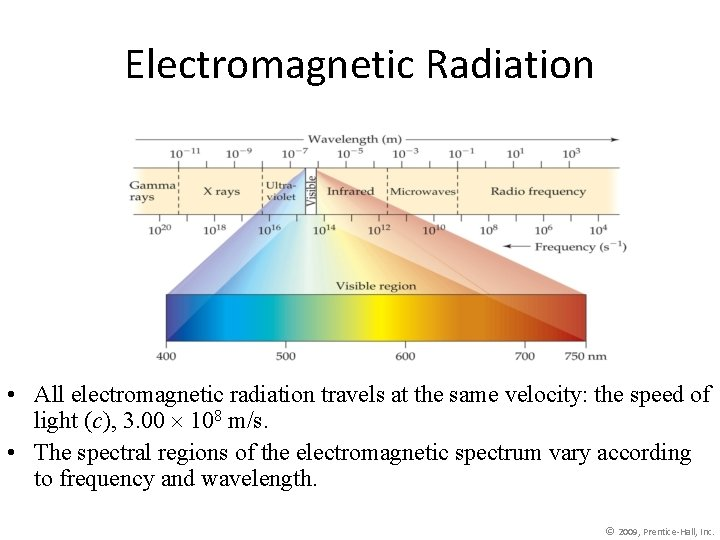Electromagnetic Radiation • All electromagnetic radiation travels at the same velocity: the speed of light (c), 3. 00 108 m/s. • The spectral regions of the electromagnetic spectrum vary according to frequency and wavelength. © 2009, Prentice-Hall, Inc.Line Spectra of Other Elements • Each element has a unique line spectrum. • The lines indicate that the electrons can only make “jumps” between allowed energy levels. • Knowing the color (wavelength) can allow us to determine the energy of the electron jumps.Formula from Equations and Constants Table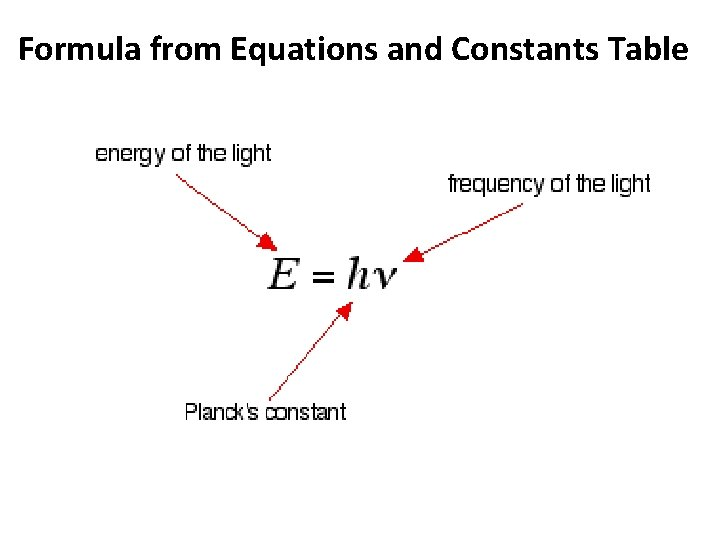Formula from Equations and Constants Table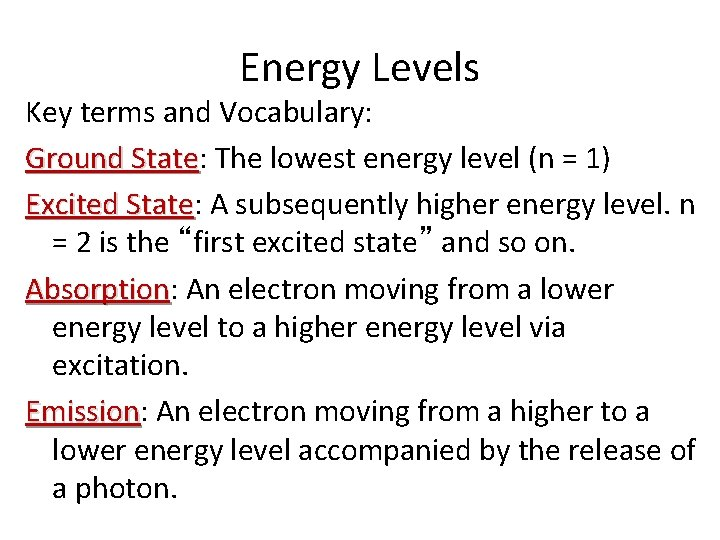Energy Levels Key terms and Vocabulary: Ground State: State The lowest energy level (n = 1) Excited State: State A subsequently higher energy level. n = 2 is the “first excited state” and so on. Absorption: Absorption An electron moving from a lower energy level to a higher energy level via excitation. Emission: Emission An electron moving from a higher to a lower energy level accompanied by the release of a photon.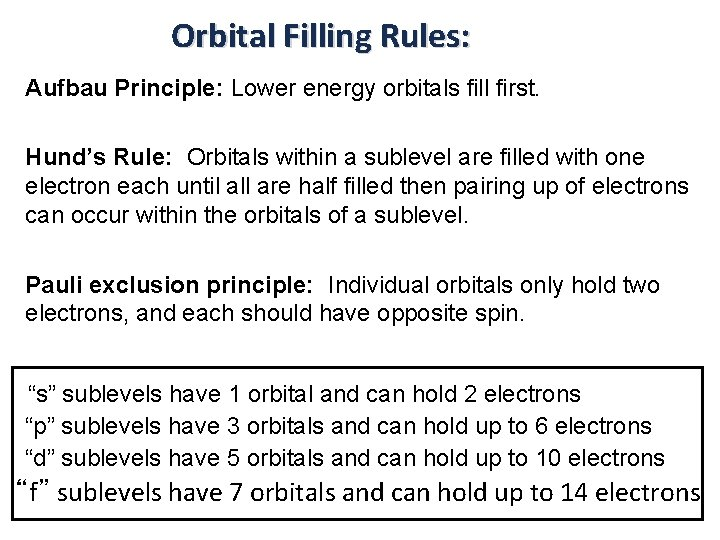Orbital Filling Rules: Aufbau Principle: Lower energy orbitals fill first. Hund’s Rule: Orbitals within a sublevel are filled with one electron each until all are half filled then pairing up of electrons can occur within the orbitals of a sublevel. Pauli exclusion principle: Individual orbitals only hold two electrons, and each should have opposite spin. “s” sublevels have 1 orbital and can hold 2 electrons “p” sublevels have 3 orbitals and can hold up to 6 electrons “d” sublevels have 5 orbitals and can hold up to 10 electrons “f” sublevels have 7 orbitals and can hold up to 14 electrons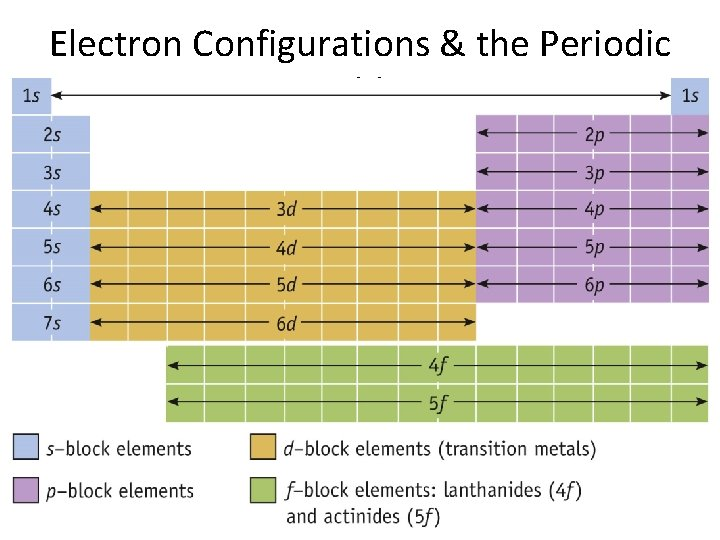Electron Configurations & the Periodic Table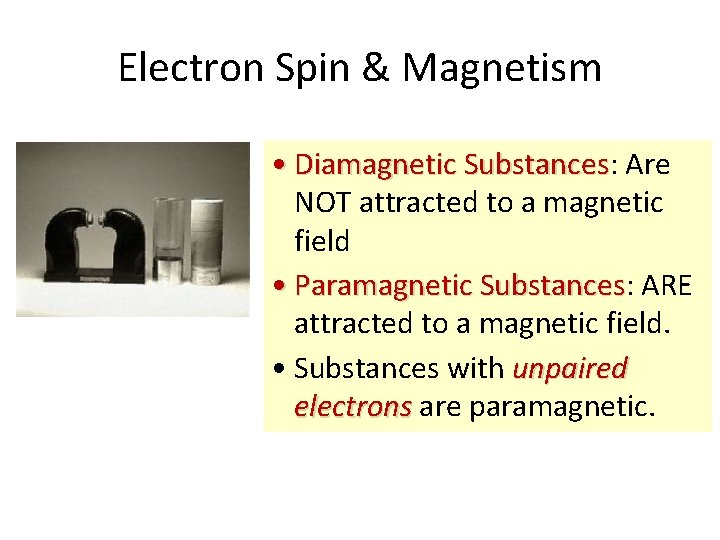Electron Spin & Magnetism • Diamagnetic Substances: Substances Are NOT attracted to a magnetic field • Paramagnetic Substances: Substances ARE attracted to a magnetic field. • Substances with unpaired electrons are paramagnetic.Measuring Paramagnetism When a substance containing unpaired electrons is place into a magnetic field, it is attracted to that magnetic field. The effect is proportional to the number of unpaired electrons.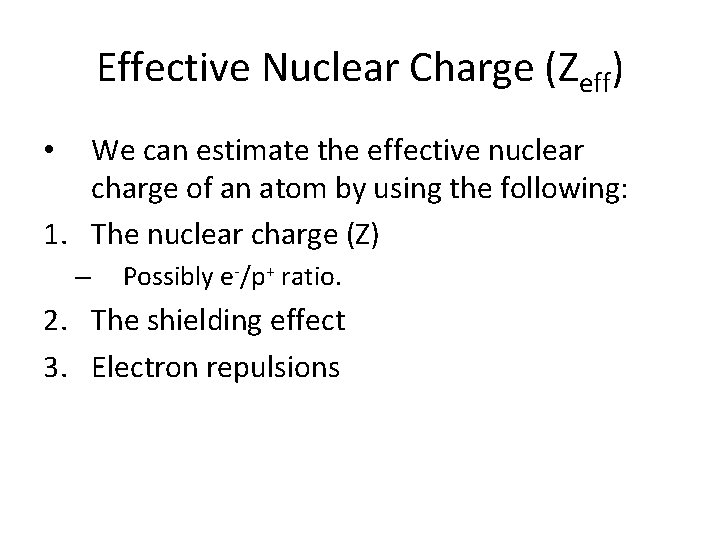Effective Nuclear Charge (Zeff) We can estimate the effective nuclear charge of an atom by using the following: 1. The nuclear charge (Z) • – Possibly e-/p+ ratio. 2. The shielding effect 3. Electron repulsionsGeneral Periodic Trends • Atomic and ionic size • Ionization energy Higher effective nuclear charge Electrons held more tightly Lower effective nuclear charge Electrons held less tightly.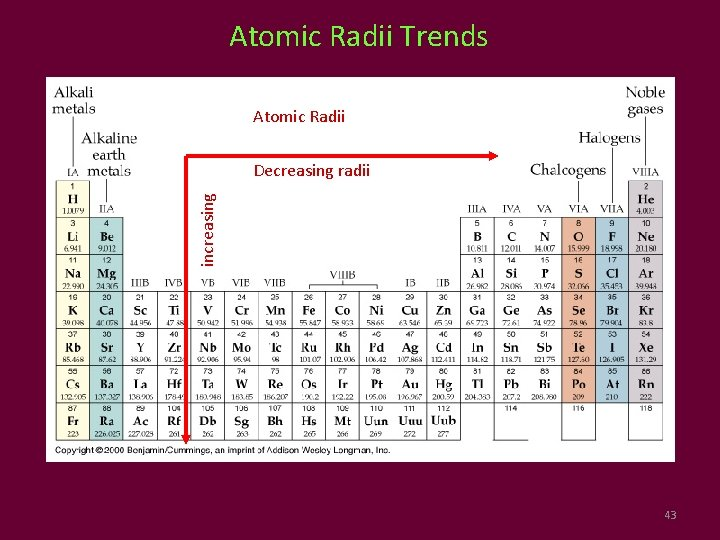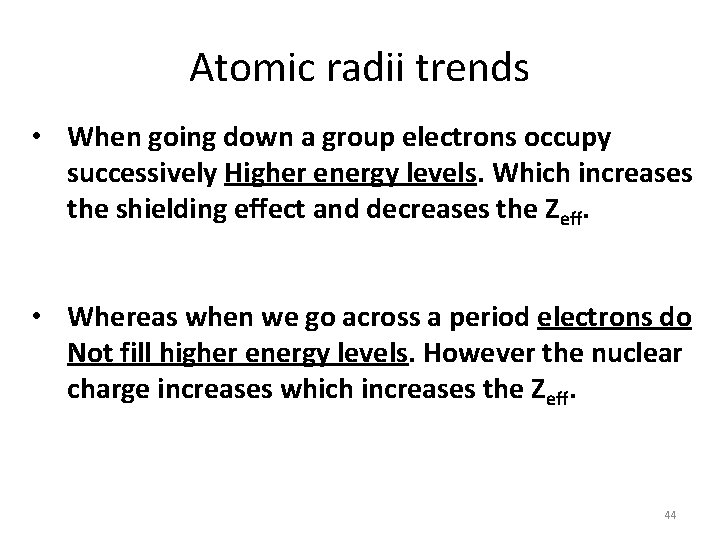Atomic radii trends • When going down a group electrons occupy successively Higher energy levels. Which increases the shielding effect and decreases the Zeff. • Whereas when we go across a period electrons do Not fill higher energy levels. However the nuclear charge increases which increases the Zeff. 44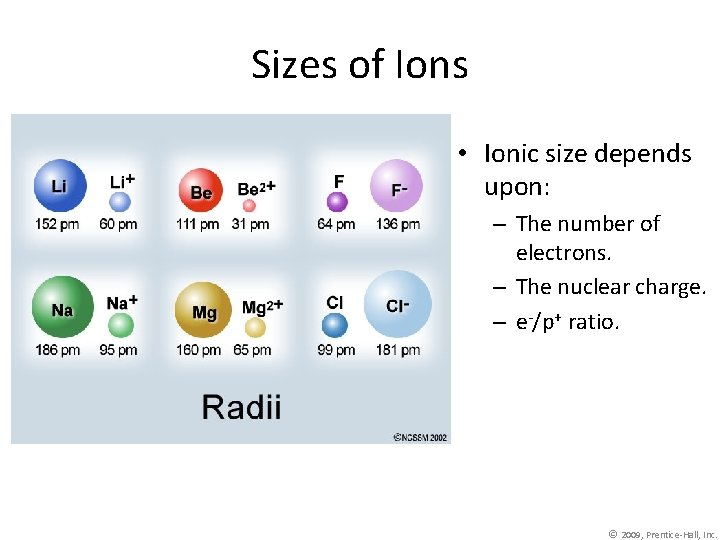Sizes of Ions • Ionic size depends upon: – The number of electrons. – The nuclear charge. – e-/p+ ratio. © 2009, Prentice-Hall, Inc.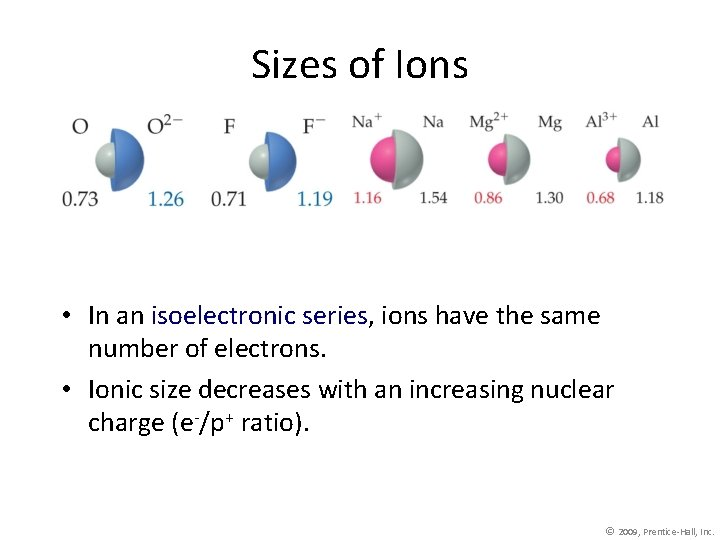Sizes of Ions • In an isoelectronic series, ions have the same number of electrons. • Ionic size decreases with an increasing nuclear charge (e-/p+ ratio). © 2009, Prentice-Hall, Inc.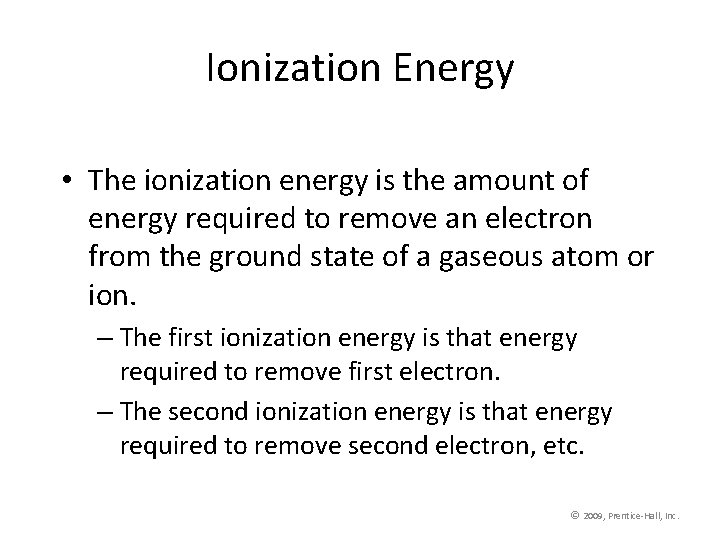Ionization Energy • The ionization energy is the amount of energy required to remove an electron from the ground state of a gaseous atom or ion. – The first ionization energy is that energy required to remove first electron. – The second ionization energy is that energy required to remove second electron, etc. © 2009, Prentice-Hall, Inc.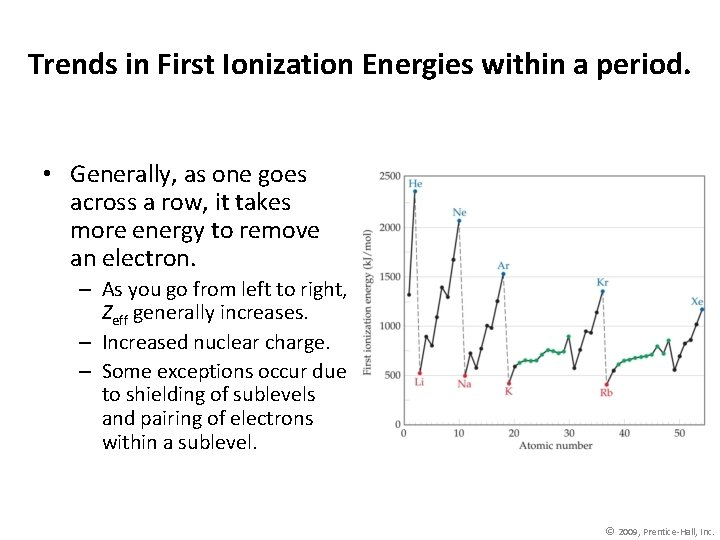Trends in First Ionization Energies within a period. • Generally, as one goes across a row, it takes more energy to remove an electron. – As you go from left to right, Zeff generally increases. – Increased nuclear charge. – Some exceptions occur due to shielding of sublevels and pairing of electrons within a sublevel. © 2009, Prentice-Hall, Inc.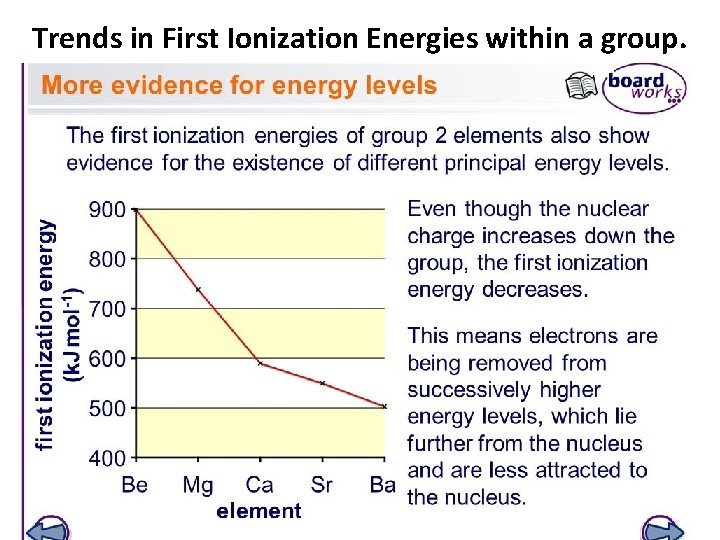Trends in First Ionization Energies within a group.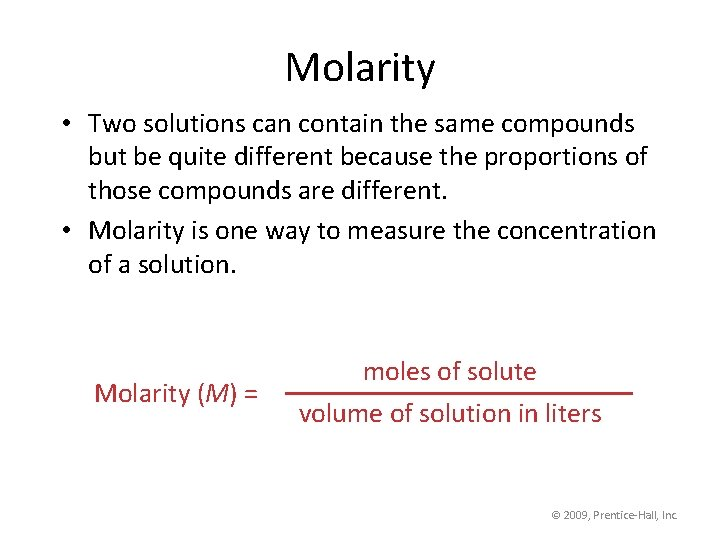Molarity • Two solutions can contain the same compounds but be quite different because the proportions of those compounds are different. • Molarity is one way to measure the concentration of a solution. Molarity (M) = moles of solute volume of solution in liters © 2009, Prentice-Hall, Inc.Assume Al 2(SO 4)3 is soluble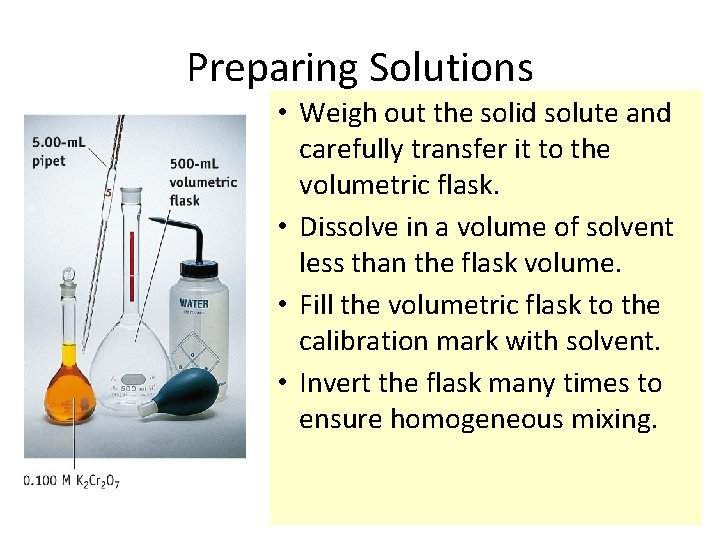Preparing Solutions • Weigh out the solid solute and carefully transfer it to the volumetric flask. • Dissolve in a volume of solvent less than the flask volume. • Fill the volumetric flask to the calibration mark with solvent. • Invert the flask many times to ensure homogeneous mixing.Preparing Solution of Known Concentration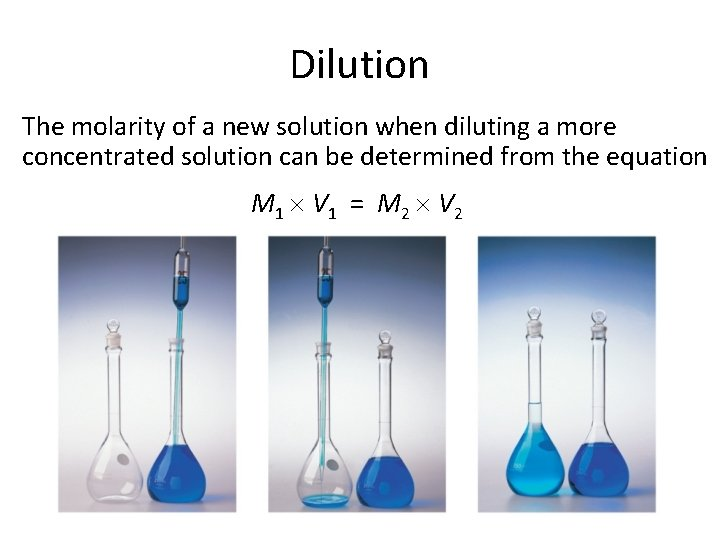Dilution The molarity of a new solution when diluting a more concentrated solution can be determined from the equation M 1 V 1 = M 2 V 2UV-Visible Spectra • Spectroscopy is the study of the interaction of energy with a sample for analysis. UV-Vis spectroscopy specifically addresses the absorbance or transmittance of a material at a specific wavelength within the UV/visible portion of the electromagnetic spectrum. • Almost every material in existence absorbs light of one wavelength or another. Materials will absorb light of one wavelength and not absorb light of a different wavelength. The absorption of specific wavelengths of light can be measured using a spectrophotometer. This information can be used to understand sample composition and concentration.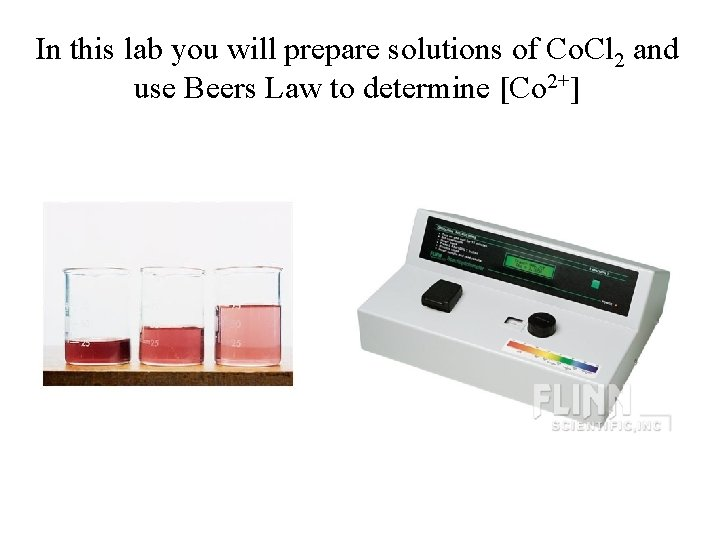In this lab you will prepare solutions of Co. Cl 2 and use Beers Law to determine [Co 2+]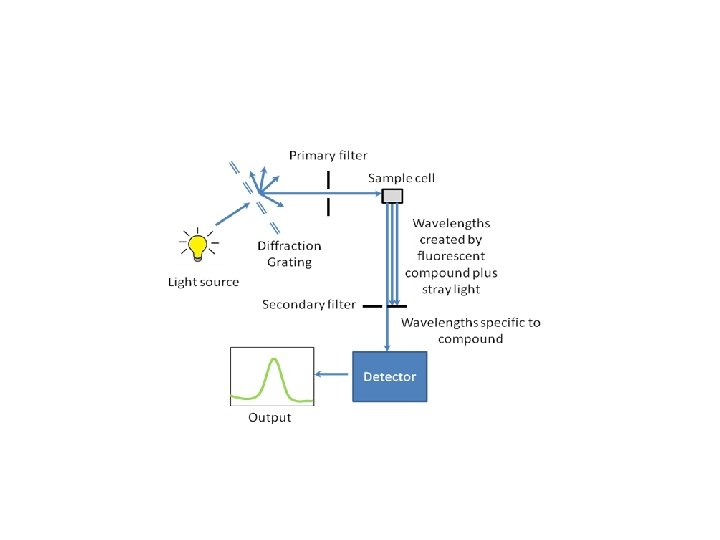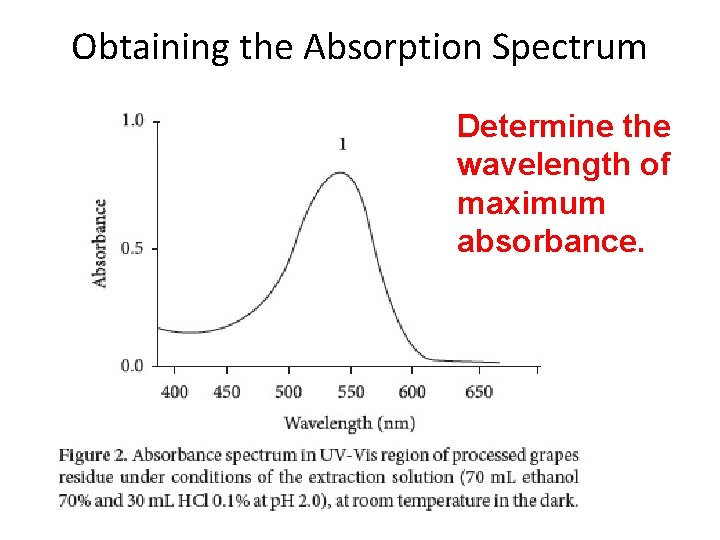Obtaining the Absorption Spectrum Determine the wavelength of maximum absorbance.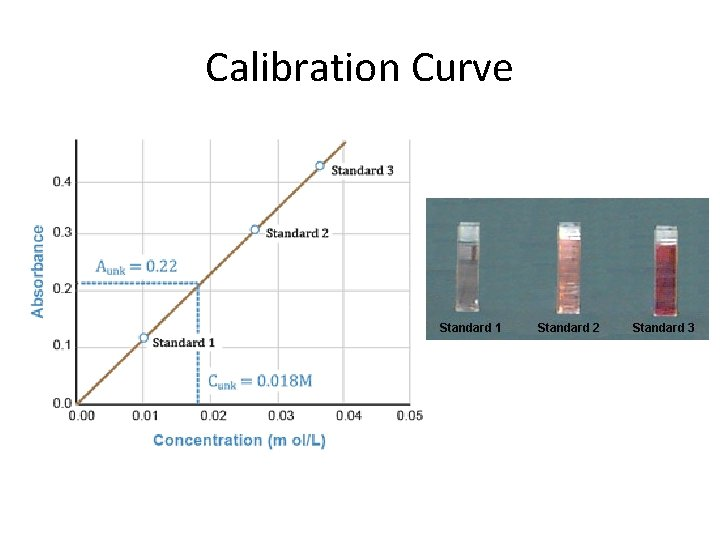Calibration Curve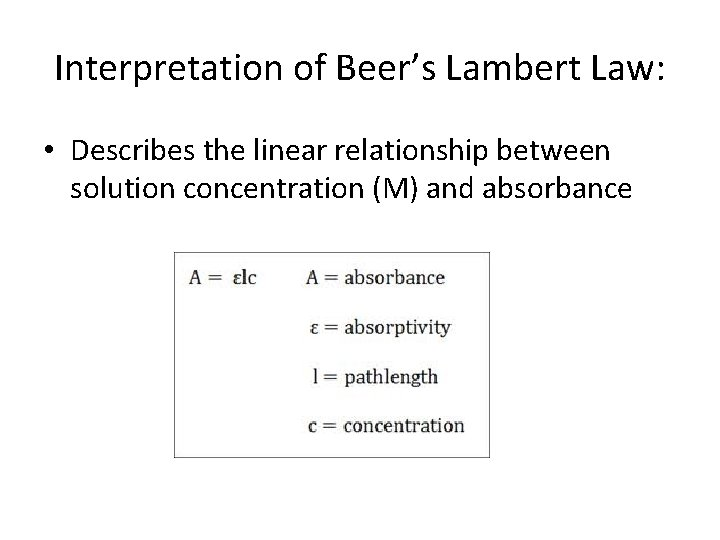Interpretation of Beer’s Lambert Law: • Describes the linear relationship between solution concentration (M) and absorbance